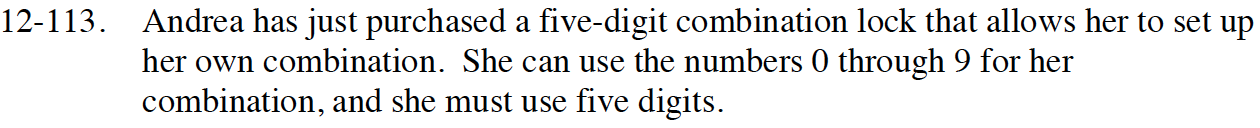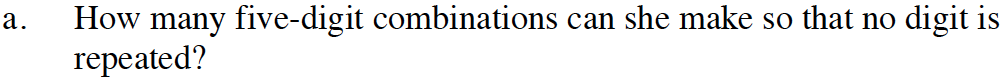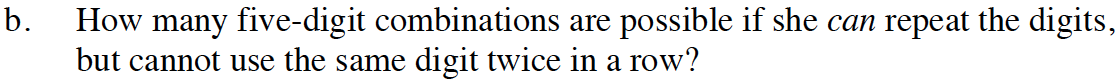Home > CCA2 > Chapter 12 > Lesson 12.2.2 > Problem12-113

12-113.
1. Andrea has just purchased a five-digit combination lock that allows her to set up her own combination. She can use the numbers 0 through 9 for her combination, and she must use five digits. Homework Help ✎

1. How many five-digit combinations can she make so that no digit is repeated?

2. How many five-digit combinations are possible if she can repeat the digits, but cannot use the same digit twice in a row?Although the problem says 'combination' this is actually a permutation
because the order you enter the numbers in the combination lock matters.Since this is a special case you can use a decision chart.
How many choices does she have for each position?

$\frac{10}{1\text{^s^t}} \frac{9}{2\text{^n^d}} \frac{9}{3\text{^r^d}} \frac{9}{4\text{^t^h}} \frac{9}{5\text{^t^h}}$